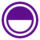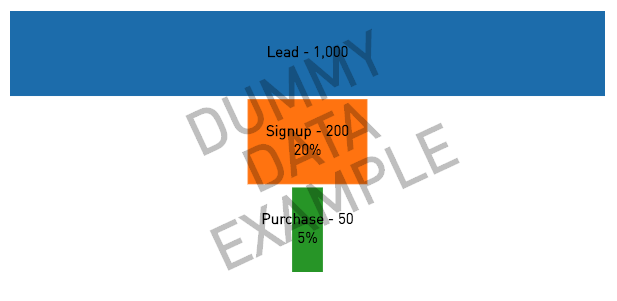# Funnel Chart — Plot.lyFunnel charts are a great way to represent any drop-offs in sample size throughout a series of steps. Using a little bit of Python handiwork in Periscope Data's R/Python integration, we can easily create this chart type.

A common use case for this chart type is to visualize your pipeline via a sales funnel. This is an extremely effective way to spot opportunities to improve your current sales process!

Below is the Python script used to create the funnel chart above and the required columns from the SQL Output:

SQL Output Requirements:

1. phase: the name of the stage in the funnel
2. value: the number of items in that stage

Python 3.6 Code

```# PERISCOPE FUNNEL CHART TEMPLATE
# SQL output should have 2 columns:
#    1) phase: the name of the stage in the funnel
#    2) value: the number of items in that stage

from __future__ import division
import pandas as pd
import plotly.plotly as py
from plotly import graph_objs as go

community_post = ''
dummy_df = pd.DataFrame()
dummy_df['PHASE'] = ['Lead', 'Signup', 'Purchase']
dummy_df['VALUE'] = ['1000', '200', '50']

# color of each funnel section
DEFAULT_PLOTLY_COLORS = [
'#1f77b4',  # muted blue
'#ff7f0e',  # safety orange
'#2ca02c',  # cooked asparagus green
'#d62728',  # brick red
'#9467bd',  # muted purple
'#8c564b',  # chestnut brown
'#e377c2',  # raspberry yogurt pink
'#7f7f7f',  # middle gray
'#bcbd22',  # curry yellow-green
'#17becf'   # blue-teal
]

def label(row, df):
label = f'<b>{row["PHASE"]}</b> - {"{:,}".format(row["VALUE"])}'
if row['INDEX'] > 0:
label += f'<br>{"{:.0%}".format(row["VALUE"]/df["VALUE"].max())}'
return label

def color(i):
return DEFAULT_PLOTLY_COLORS[i % len(DEFAULT_PLOTLY_COLORS)]

style = ';'.join([f'{key.replace("_","-")}:{settings[key]}' for key in settings])
return f'<a href="{link}" style="{style}">{text}</a>'

def plot(df, annotation=None):
df.columns = [c.upper() for c in df.columns]
df['VALUE'] = pd.to_numeric(df['VALUE'])
df['INDEX'] = df.index
df['LABELS'] = df.apply(lambda row: label(row, df), axis=1)

# chart stages data
values = df['VALUE']
phases = df['PHASE']

n_phase = len(phases)
plot_width = 400

# height of a section and difference between sections
section_h = 20
section_d = 1

# multiplication factor to calculate the width of other sections
unit_width = plot_width / max(values)

# width of each funnel section relative to the plot width
phase_w = [int(value * unit_width) for value in values]

# plot height based on the number of sections and the gap in between them
height = section_h * n_phase + section_d * (n_phase - 1)

# list containing all the plot shapes
shapes = []

# list containing the Y-axis location for each section's name and value text
label_y = []

for i in range(n_phase):
if (i == n_phase-1):
points = [phase_w[i] / 2, height, phase_w[i] / 2, height - section_h]
else:
points = [phase_w[i] / 2, height, phase_w[i+1] / 2, height - section_h]
print(points)
path = 'M {0} {1} L {0} {3} L -{0} {3} L -{0} {1} Z'.format(*points)

shape = {
'type': 'path',
'path': path,
'fillcolor': color(i),
'line': {
'width': 1,
'color': color(i)
},
'layer': 'below'
}
shapes.append(shape)

# Y-axis location for this section's details (text)
label_y.append(height - (section_h / 2))

height = height - (section_h + section_d)

# For phase names
label_trace = go.Scatter(
x=*n_phase,
y=label_y,
mode='text',
text=df['LABELS'],
textfont=dict(
color='#000000',
size=15
),
hoverinfo='text'
)

data = [label_trace]

layout = go.Layout(
#     title="<b>Funnel Chart</b>",
titlefont=dict(
size=20,
color='rgb(203,203,203)'
),
hovermode = 'closest',
shapes=shapes,
#     height=560,
#     width=800,
showlegend=False,
#     paper_bgcolor='rgba(44,58,71,1)',
#     plot_bgcolor='rgba(44,58,71,1)',
xaxis=dict(
showticklabels=False,
zeroline=False,
showgrid=False,
ticks=''
),
yaxis=dict(
showticklabels=False,
zeroline=False,
showgrid=False,
ticks=''
),
margin=dict(
t=20,
b=50,
l=10,
r=10
)
)

if annotation is not None:
layout['annotations'] = [annotation]

fig = go.Figure(data=data, layout=layout)

# For Python 2 & 3, pass configs into plotly (i.e `plotly_output(figure,config={'displayModeBar':True})
periscope.plotly(fig, config={'displayModeBar':False})

try:
i = int('e')
plot(df)
except Exception as e:
print(e)
annotation = {
'x': 0.5,
'y': 0.5,
'ax': 0,
'ay': 0,
'xref': 'paper',
'yref': 'paper',
'text': style_link('DUMMY<br><br><br><br>DATA<br><br><br><br>EXAMPLE', community_post, font_size='60px', font_weight='bold', color='rgba(0, 0, 0, .25)'),
'showarrow': False,
'textangle': -25
}
plot(dummy_df, annotation=annotation)```

Some more examples of funnel charts: